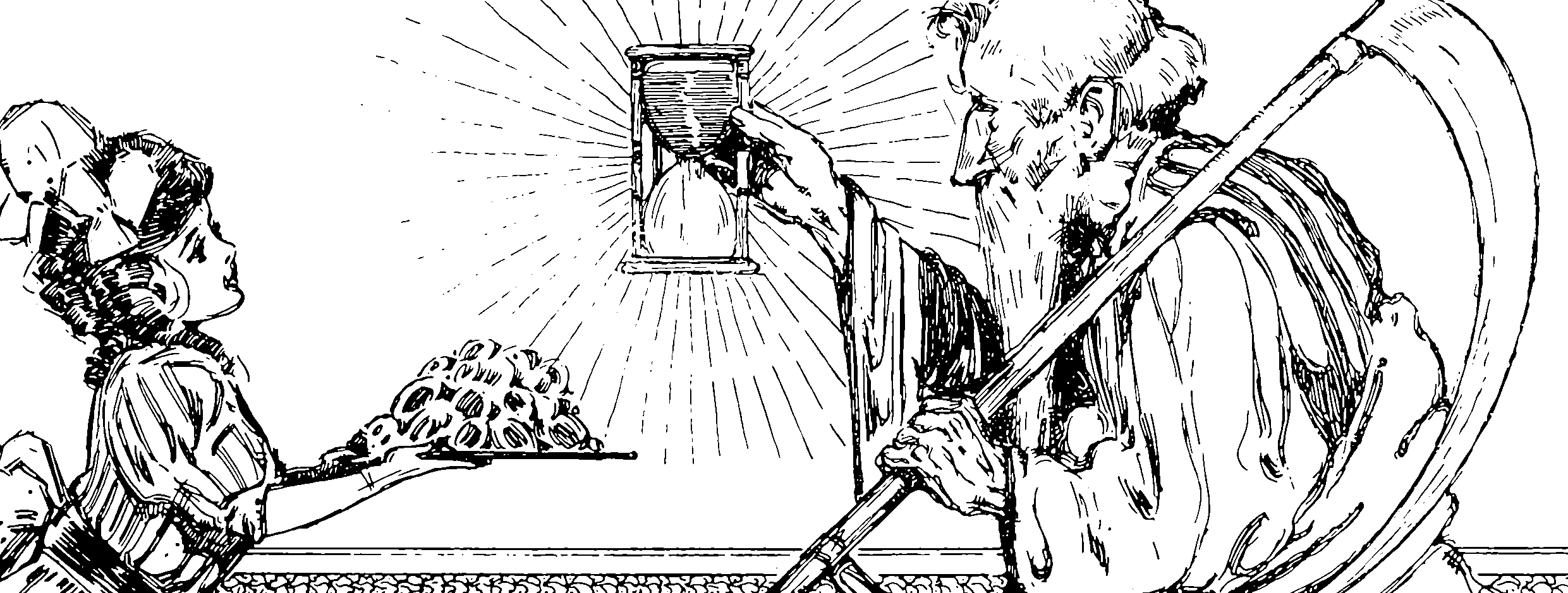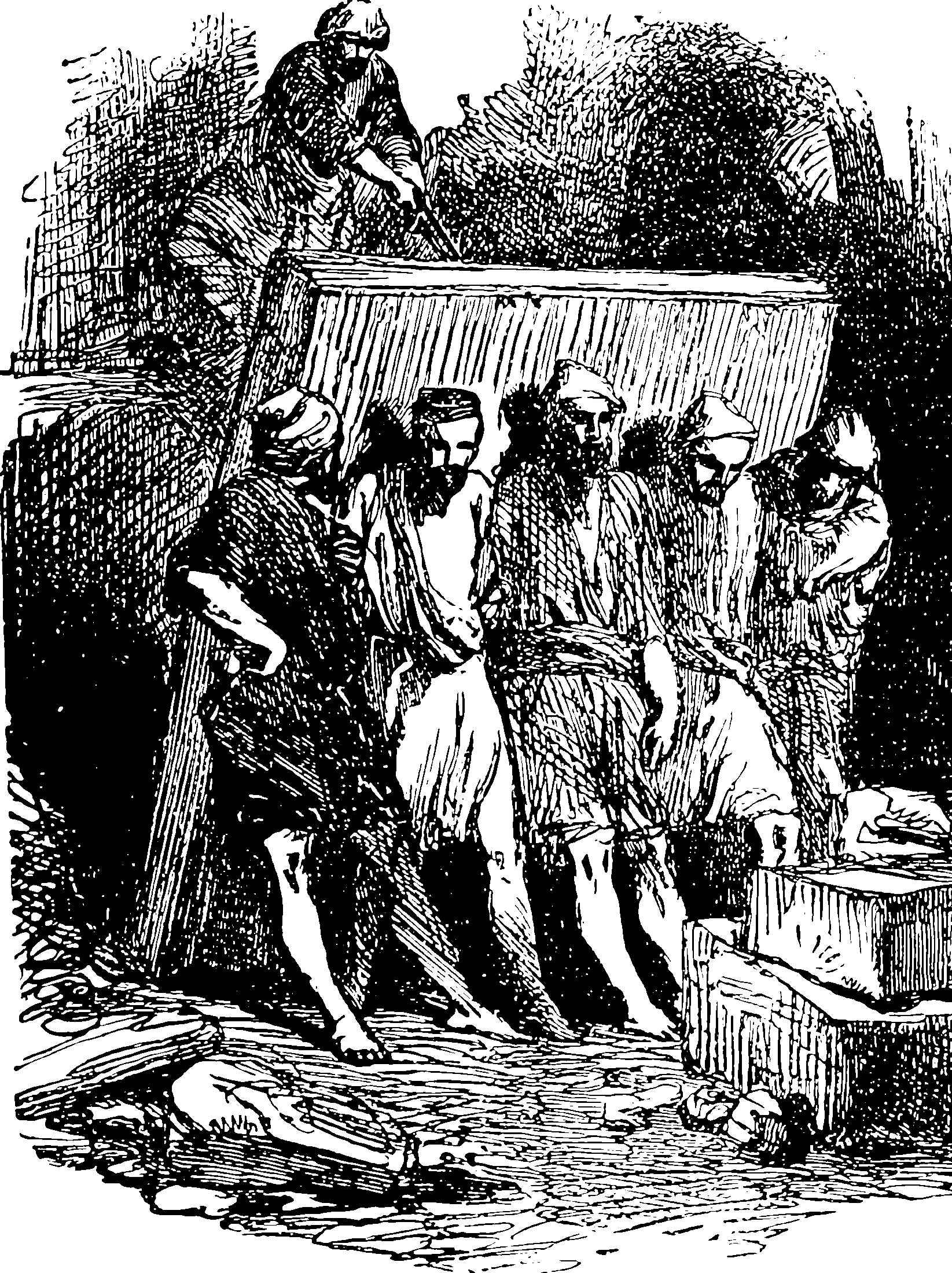# The edge of chaos

## Computation, evolution, competition and other past-times of facultyI did not create an edge-of-chaos notebook for a while because I could not face the task of sifting through the woo-woo silt to find the gold. There is some gold, though, and also some iron pyrites which is still nice to look at, and some fun ideas, and also a wacky story of cranks, mavericks and Nobel prizes and hype which is interesting in its own right.

Name check: criticality, which is also perhaps related to dynamical stability, and ergodicity, and inevitably to fractals because of scaling relations. Somewhere out of this, a theory of algorithmic statistics might emerge.

Maybe information bottlenecks also?

## History

Start with Crutchﬁeld and Young (1988);Chris G. Langton (1990) which introduce the association between the edge of chaos and computing.

## Which chaos? Which edge?

Two ingredients seem to be popular: a phase transition model and an assumed computation model. TBD

## In lifeTBD.

## Neural nets

A resurgence of interest in edge of chaos is in the undifferentiated, random computational mess of neural networks, which do look in fact a lot like a system which should demonstrate edge-of-chaos computation if anything does. See also statistical mechanics of statistics.

Hayou et al. (2020) argues that deep networks are trainable in an edge-of-chaos context, building on earlier work . There is a very specific notion of chaos here, which looks like one about measure-preserving, mixing dynamical systems. To revisit.

Roberts, Yaida, and Hanin (2021) may or may not relate:

This book develops an effective theory approach to understanding deep neural networks of practical relevance. Beginning from a first-principles component-level picture of networks, we explain how to determine an accurate description of the output of trained networks by solving layer-to-layer iteration equations and nonlinear learning dynamics. A main result is that the predictions of networks are described by nearly-Gaussian distributions, with the depth-to-width aspect ratio of the network controlling the deviations from the infinite-width Gaussian description. We explain how these effectively-deep networks learn nontrivial representations from training and more broadly analyze the mechanism of representation learning for nonlinear models. From a nearly-kernel-methods perspective, we find that the dependence of such models' predictions on the underlying learning algorithm can be expressed in a simple and universal way. To obtain these results, we develop the notion of representation group flow (RG flow) to characterize the propagation of signals through the network. By tuning networks to criticality, we give a practical solution to the exploding and vanishing gradient problem. We further explain how RG flow leads to near-universal behavior and lets us categorize networks built from different activation functions into universality classes. Altogether, we show that the depth-to-width ratio governs the effective model complexity of the ensemble of trained networks. By using information-theoretic techniques, we estimate the optimal aspect ratio at which we expect the network to be practically most useful and show how residual connections can be used to push this scale to arbitrary depths. With these tools, we can learn in detail about the inductive bias of architectures, hyperparameters, and optimizers.

### No comments yet. Why not leave one?

GitHub-flavored Markdown & a sane subset of HTML is supported.